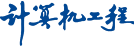##• 开发研究与工程应用 •

### 基于结点间距离统计的无向无权图同构判别

1. (广东工业大学计算机学院，广州 510006)
• 收稿日期:2012-06-12 出版日期:2013-06-15 发布日期:2013-06-14
• 作者简介:陈伟平(1987－)，男，硕士研究生，主研方向：组合图论；战荫伟，教授、博士

### Undirected and Unweighted Graph Isomorphism Determination Based on Distance Statistics of Vertices

CHEN Wei-ping, ZHAN Yin-wei

1. (Faculty of Computer, Guangdong University of Technology, Guangzhou 510006, China)
• Received:2012-06-12 Online:2013-06-15 Published:2013-06-14

Abstract: To determine two graphs are isomorphic or not by the definition of graph isomorphism, at worst, the time complexity is O(N!). Against this problem, it introduces an algorithm which is based on the distance statistics of the vertices. Vertices are stratified by distance, by counting the numbers of related edges and related vertices of vertices which are isometric. The algorithm shows the difference of the vertices, and points out the vertices correspondence of two graphs. If the vertices distance array and layer related vertices array of two graphs are not one-to-one, the time complexity of the algorithm is only O(N4). If they are one-to-one, swap the sequence of the vertices in one graph which may correspond to the vertex in the other graph, avoid swapping the sequence of all vertices, in this condition, the time complexity is reduced by many times.